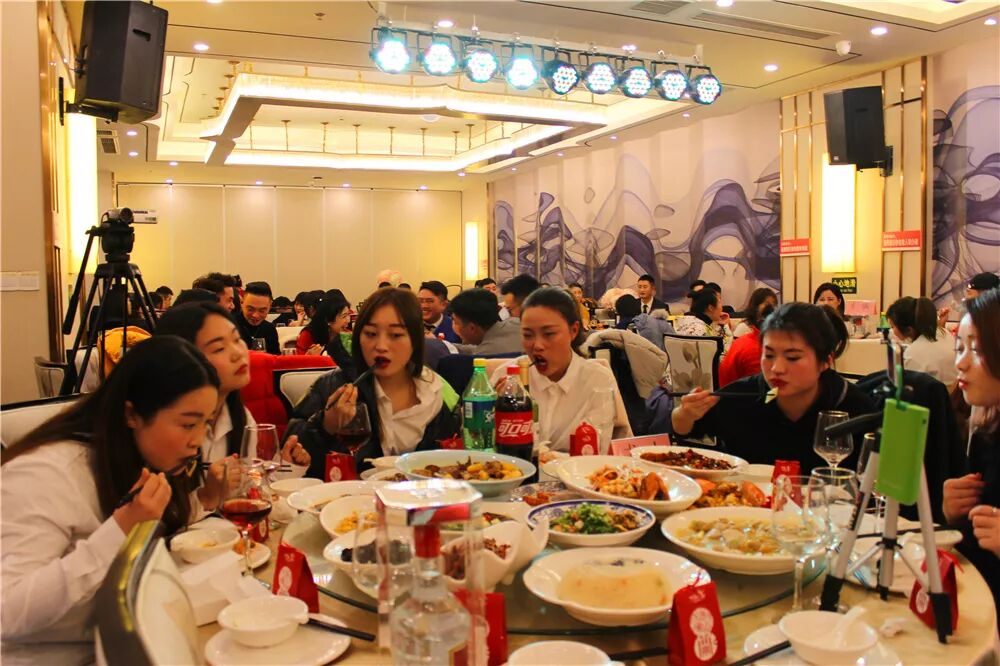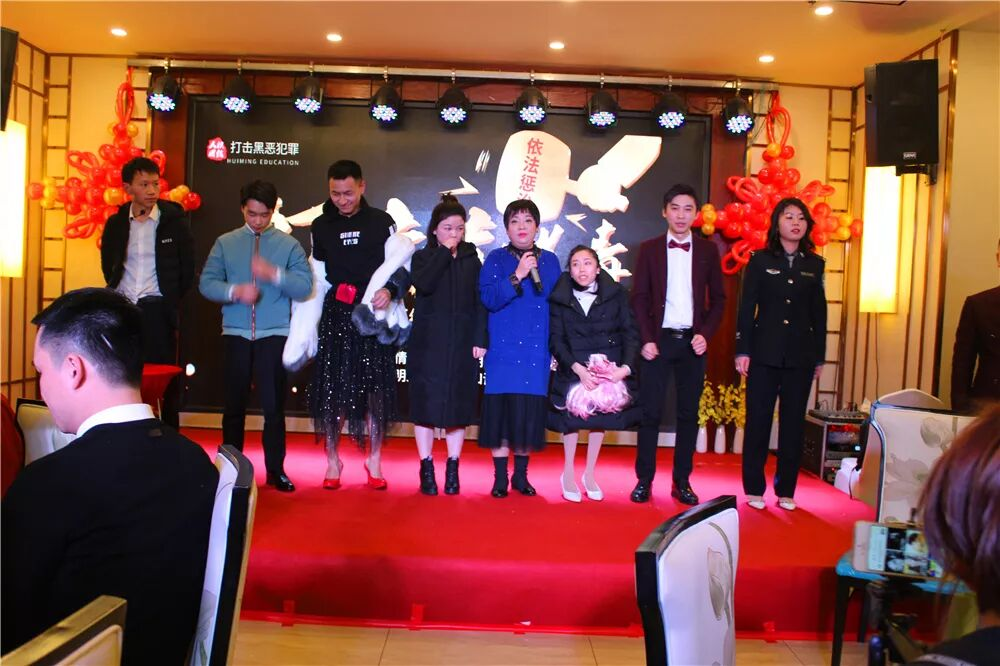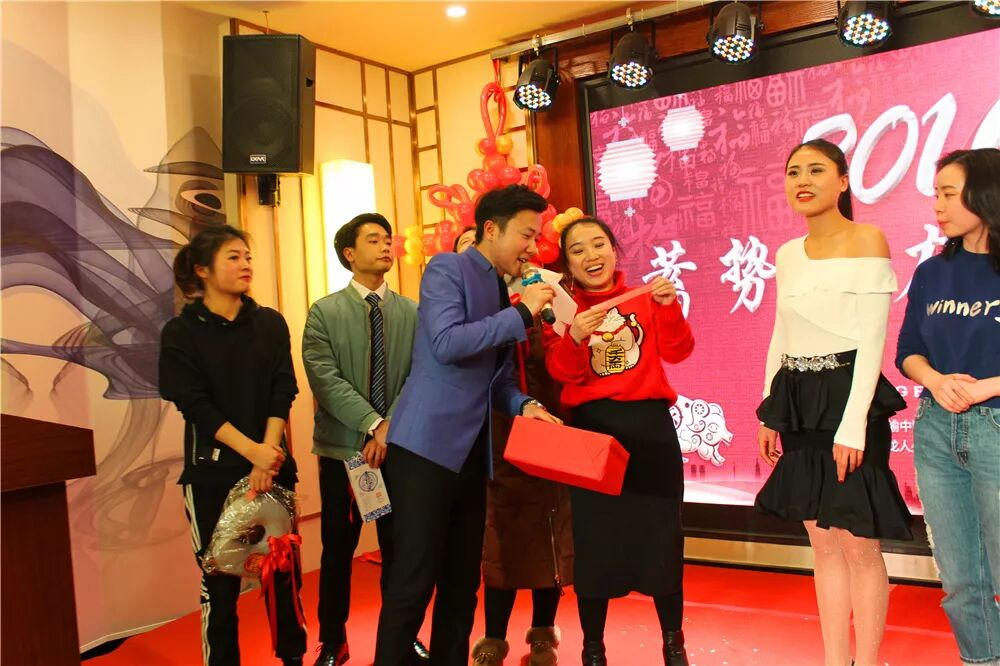最新消息：

# 蓄势聚力，共赴辉煌 | 2019汇名年度盛典感恩有你相伴<<  滑动查看下一张图片  >><<  滑动查看下一张图片  >><<  滑动查看下一张图片  >><<  滑动查看下一张图片  >><<  滑动查看下一张图片  >><<  滑动查看下一张图片  >><<  滑动查看下一张图片  >>新的一年开启新的希望
<section powered-by="xiumi.us" helvetica="" neue",="" "pingfang="" sc",="" "hiragino="" sans="" gb",="" "microsoft="" yahei="" ui",="" yahei",="" arial,="" sans-serif;="" font-size:="" 16px;="" font-variant-numeric:="" normal;="" font-variant-east-asian:="" letter-spacing:="" 0.544px;="" line-height:="" 25.6px;="" text-align:="" justify;="" widows:="" 1;="" overflow-wrap:="" break-word="" !important;"="" style="margin: 0px; padding: 0px; max-width: 100%; box-sizing: border-box; color: rgb(51, 51, 51);">

### 学员风采more

#### 婚礼主持人培训第75期学员QQ在线咨询